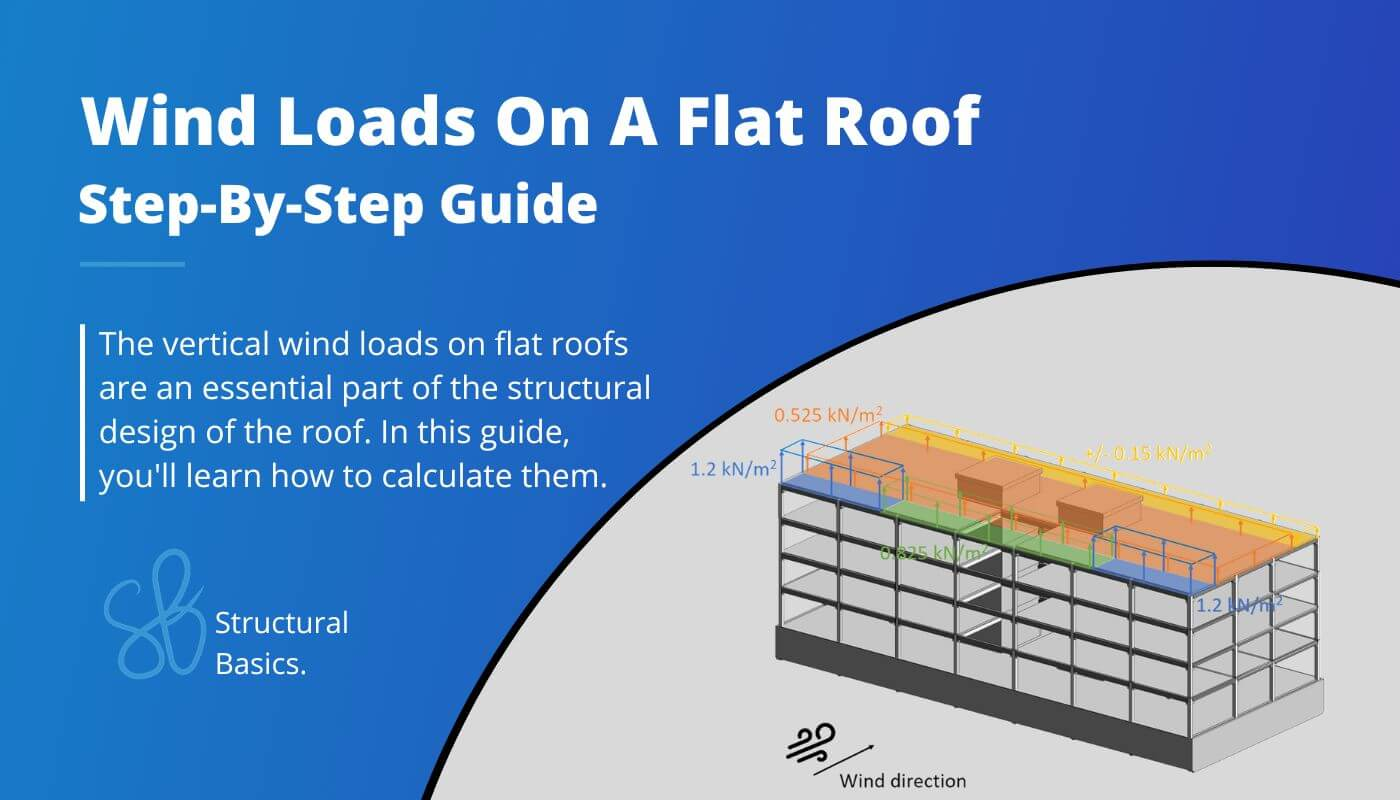# How To Calculate The Wind Loads Of A Flat RoofIn this article we are going to calculate the wind load on flat roofs according to EN 1991-1-4:2005. As we already did for the wind load calculation of the walls/facades, we are going to explain it with an example of a precast concrete office building, which you can see in the next figure. The following picture shows only the structural elements.

The calculation of the wind load is split up in multiple articles due to the fact that the wind load depends on much more parameters that need to be derived than for example the snow load.

In order to calculate the wind load or wind pressure on external surfaces of the flat roof, we are going to do the following steps:

1. Calculate the wind velocity pressure $q_{p}$
2. Define the outer geometry of the building
3. Calculate the width of the Wind areas
4. Find the external pressure coefficients

## Geometry and parameters from wind velocity pressure

This is a quick summary of the values we calculated to get the wind velocity pressure.

## Wind pressure on surfaces

### Wind pressure on external surfaces $w_{e}$

The formula (EN 1991-1-4:2005 (5.1)) to calculate the wind pressure on external surfaces is

$w_{e} = q_{p} * c_{pe}$

Where

The coefficient $c_{pe}$ has 2 different values depending on the wind loaded area. There is a value for a surface area of 1 $m^2$ and 10 $m^2$. These two values can also be written as

We explained it already a bit more detailed here. If you want to a more detailed explanation then either go to the article or read up in EN 1991-1-4:2005 7.2.

EN 1991-1-4 Table 7.2 gives recommendations for $c_{pe.10}$ and $c_{pe.1}$.

❗This means that you have to double-check with your National annex because those values might be defined differently there❗

Table 7.2 gives values for 4 different areas F, G, H and I of our building.

Those areas depend on where the wind comes from and can be seen in EN 1991-1-4:2005 Table 7.2. For our office building, we can define the areas as

### Wind from Front

From those dimensions we can define $e$ which determines the widths and depths of Areas F, G and H according to EN 1991-1-4:2005 Figure 7.6

$e = min(b, 2h)$

$e = min(49.75m, 2*17.1m=34.4m) = 34.4m$

From e we get the dimensions of the areas according to EN 1991-1-4:2005 Figure 7.6

So many numbers – I know. Let’s visualize them 😁

Okay, now let’s go back to the formula for the wind pressure for external surfaces and derive the values. The peak velocity pressure was calculated (in the last article) as:

$q_{p} = 0.746 \frac{kN}{m^2}$

Now, the external pressure coefficient also depends on the roof type. The following roof types are included:

• Sharp eaves
• With parapets
• Curved eaves and
• Mansard eaves

For the roof type of parapet with the parapet height $h_{p}$

$h_{p} = 50cm$

we calculate

$h_{p}/h = 0.5m/17.1m = 0.03$

to then look up the external pressure coefficients for our Areas.

The external pressure coefficients for rectangular buildings can be taken from EN 1991-1-4:2005 Table 7.2.

Don’t be confused by the + and – of the coefficients of Area I. EN 1991-1-4:2005 Table 7.2 Note 3 says that in Area I both positive and negative value should be considered.

Based on our coefficients, we can now calculate the Wind pressure on external surfaces.

When you calculate the wind loads the first time ever, it might be very confusing in which direction you have to apply the loads. So let’s apply the wind loads with the $c_{pe.10}$ coefficient (for 10 $m^2$) on our building.

Now we also have to do the same for the case that wind comes from the side.

### Wind from Side

In the scenario that wind comes from the side, we have to define the Area widths again. We have to redefine the geometry parameters.

From those dimensions we can define $e$ which determines the widths and depths of Areas F, G and H according to EN 1991-1-4:2005 Figure 7.6

$e = min(b, 2h)$

$e = min(49.75m, 2*17.1m=34.4m) = 34.4m$

From e we get the dimensions of the areas according to EN 1991-1-4:2005 Figure 7.6

As we all know, a picture tells more than a thousand words – so let’s visualize all of those numbers 😁

For the roof type of a parapet with the parapet height ratio $h_{p}/h = 0.03$ we can look up the external pressure coefficients for rectangular buildings in EN 1991-1-4:2005 Table 7.2

Based on our coefficients, we can now calculate the Wind pressure on external surfaces.

Those wind area loads we can now visualize.